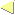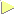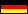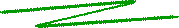Quantum LogicThe basis for quantum computation is not Boolean logic, but quantum logic. To date, there is still no appropriate quantum-logical calculus comparable to the classical calculus based on Boolean algebra. There are at least two essential differences between quantum and Boolean logic. One is that any quantum gate has to be reversible, i.e., input and output must always correspond uniquely to one another. In particular, the number of input and output qubits have to be equal. This is different than in the Boolean case, where most gates have two input bits and only one output bit. In fact, all basic binary operations of Boolean algebra (∧, ∨, ¬, XOR, NAND, NOR, ...) are 2-1 valued, which implies that they are not reversible: in fact, since 1 ∧ 0 = 0 ∧ 1 = 0 ∧ 0 = 0, you cannot deduce from the result “0” which values the input bits have had.3

Another difference between quantum and Boolean logic is that quantum gates can transform a qubit basis, say {|0>, |1>}, to another, for instance {|0> + |1>, |0> - |1>}, just as a vector basis can be changed by reflections or rotations. This property is impossible in Boolean logic, where any operation transforms to one of the two values 0 or 1. In other words, the basis is never changed in Boolean logic.

 © de Vries 2004 – 2012